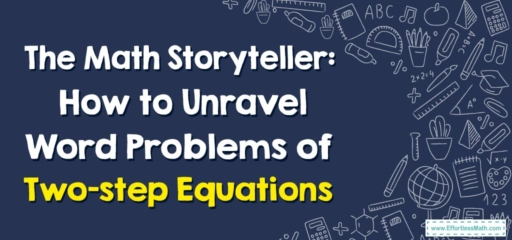# The Math Storyteller: How to Unravel Word Problems of Two-step Equations

Today, we embark on a new quest: unraveling word problems of two-step equations. Just like reading a story, solving word problems involves understanding the plot and untangling the details to find our answer. Let's set forth on our journey!## 1. The Characters: Two-step Equations

In our stories, two-step equations play a leading role. These equations take us on a journey of solving for an unknown, step by step.

## 2. The Plot: Word Problems

The word problems set the stage for our adventure. They describe a scenario that we need to translate into a two-step equation and solve.

Let’s delve into the journey:

#### Step 1: Understand the Problem

Read the problem carefully, just like you would a story. What is happening? What do we need to find?

#### Step 2: Translate the Words into an Equation

Take the scenario and turn it into a two-step equation. Look for clues in the problem that indicate mathematical operations.

#### Step 3: Solve the Equation

Apply the two-step equation-solving skills we learned previously to find the unknown.

Consider this example: Jenny saves $$15$$ from her allowance every week. After $$5$$ weeks, she has $$95$$. How much money did Jenny start with?

1. Understand the Problem: We need to find how much money Jenny started with. She saves $$15$$ every week for $$5$$ weeks, and now she has $$95$$.
2. Translate the Words into an Equation: If Jenny started with x dollars, then according to the problem, $$x + 15\times 5 = 95$$.
3. Solve the Equation: We solve this two-step equation to find that $$x = 20$$.

And thus, our adventure concludes! With each word problem, we’re not just solving equations – we’re unraveling a math story. Practice makes perfect, so keep going on these math adventures and soon you’ll become the ultimate math storyteller. Until our next adventure, happy problem-solving!

### What people say about "The Math Storyteller: How to Unravel Word Problems of Two-step Equations - Effortless Math: We Help Students Learn to LOVE Mathematics"?

No one replied yet.

X
30% OFF

Limited time only!

Save Over 30%

SAVE $5 It was$16.99 now it is \$11.99